# Oil Material Balance

## Brief

The general form of the Oil Material Balance equation was first published by Schilthuis in 1941.

## Math & Physics

Equating all underground withdrawals to the sum of the volume changes: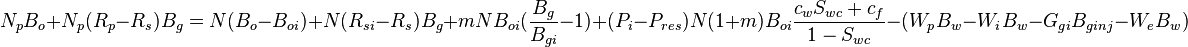$N_p B_o + N_p (R_p - R_s) B_g = N (B_o - B_{oi}) + N (R_{si} - R_s) B_g + m N B_{oi} (\frac{B_g}{B_{gi}} - 1) + (P_i - P_{res}) N (1 + m) B_{oi} \frac{c_w S_{wc} + c_f}{1 - S_{wc}}- (W_p B_w - W_i B_w - G_{gi} B_{ginj} - W_e B_w)$

For use in the code to find Pres:

Pres = Pi - (Np * Bo + Np * (Rp - Rs) * Bg + (Wp * Bw - Wi * Bw - Ggi * Bginj - We * Bw) - (N * (Bo - Boi) + N * (Rsi - Rs) * Bg + m * N * Boi * (Bg / Bgi - 1))) * (1 - Swc) / (N * (1 + m) * Boi * (cw * Swc + cf))


For use in the code to find Np:

Np = (N * (Bo - Boi) + N * (Rsi - Rs) * Bg + m * N * Boi * (Bg / Bgi - 1) + N * (1 + m) * Boi * (Pi - Pres) * (cw * Swc + cf) / (1 - Swc) - (Wp * Bw - Wi * Bw - Gging * Bgi - We * Bw)) / (Bo + (Rp - Rs) * Bg)


## Discussion

... most powerful tool for investigating reservoirs and understanding their performance ...
— L.P. Dake 
... the safest technique in the business since it's minimum assumption route through the subject of reservoir engineering ...
— L.P. Dake 

## Nomenclature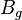$B_{g}$ = gas formation volume factor at Pres, bbl/scf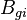$B_{gi}$ = initial gas formation volume factor, bbl/scf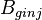$B_{ginj}$ = injection gas formation volume factor at Pres, bbl/scf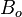$B_o$ = oil formation volume factor at Pres, bbl/stb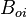$B_{oi}$ = initial oil formation volume factor, bbl/stb$B_w$ = water formation volume factor at Pres, bbl/stb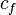$c_f$ = formation compressibility at initial pressure and temperature, 1/psia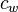$c_w$ = water compressibility at Pres, 1/psia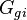$G_{gi}$ = gas injection volume, scf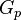$G_p$ = gas cumulative production volume, scf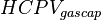$HCPV_{gascap}$ = initial gas cap hydrocarbon pore volume, bbl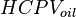$HCPV_{oil}$ = initial oil hydrocarbon pore volume, bbl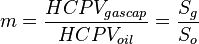$m = \frac{HCPV_{gascap}}{HCPV_{oil}}=\frac{S_g}{S_o}$ , initial gas cap oil leg ratio, dimensionless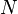$N$ = stock tank oil initially in place, stb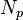$N_p$ = oil cumulative production volume, stb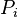$P_i$ = initial reservoir pressure, psia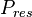$P_{res}$ = average reservoir pressure, psia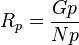$R_p = \frac{Gp}{Np}$ , cumulative GOR, scf/stb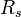$R_s$ = solution oil-gas ratio, scf/bbl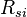$R_{si}$ = initial solution oil-gas ratio, scf/bbl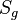$S_{g}$ = initial gas saturation, fraction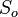$S_{o}$ = initial oil saturation, fraction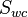$S_{wc}$ = connate water saturation, fraction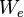$W_e$ = water influx volume, stb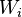$W_i$ = water injection volume, stb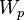$W_p$ = water production volume, stb